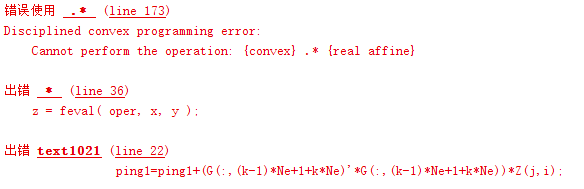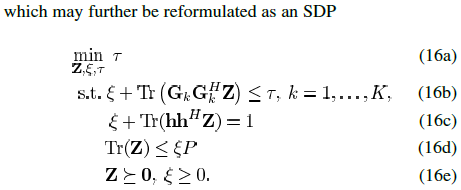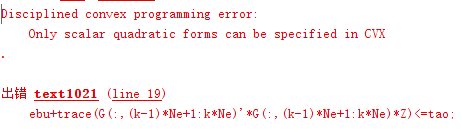# How can I express 'a+trace(A*A^H*X)' in cvx?

‘a’ is a scalar, ‘A’ is a matrix, ‘A^H’ is Hermitian transmit of ‘A’, and ‘X’ is a matrix. ‘a’ and ‘A’ are all given variables.

By “given” variables, I assume you mean input data , rather than CVX (optimization) variables. So I am assuming`X` is the only optimization variables in this expression.

Given that, everything is affine, so you can enter it “as is”:

`a + trace(A*A'*X)`

Thank you,Mark

now, my confusion is this:
Disciplined convex programming error:
Cannot perform the operation: {convex} .* {real affine}

The code is:

``````E=2;
Nt=64;
Ne=4;
ping1=0;
ping2=0;
G=randn(Ne,Nt*E)+1i*randn(Ne,Nt*E);
h=randn(1,Nt)+1i*randn(1,Nt);
Z=randn(Nt,Nt)+1i*0;
cvx_begin sdp quiet
variable tao(1,1) nonnegative
variable ebu(1,1) nonnegative
variable G(Ne,Nt*E) complex
variable Z(Nt,Nt) complex semidefinite
variable h(1,Nt) complex
variable ping(1,1)
minimize(tao)
subject to
%trace(conj(Gk.')*Gk*Z)=sum ij(conj(Gk(:,i).')*Gk(:,j)*Z(j,i))
for k=1:E
for i=1:Nt
for j=1:Nt
ping1=ping1+(G(:,(k-1)*Ne+1+k*Ne)'*G(:,(k-1)*Ne+1+k*Ne))*Z(j,i);
end
end
ebu+ping1 <= tao;
end

%trace(conj(h.')*h*Z)=sum ij(conj(h(i).')*h(j)*Z(j,i))
for i=1:Nt
for j=1:Nt
ping2=ping2+(h(i)'*h(j))*Z(j,i);
end
end
ebu+ping2 == 1;
trace(Z) <= ebu*P;
Z >= 0
ebu >= 0;
``````

cvx_end`ping1` and `ping2` are both non-convex.

‘ping1’ and ‘ping2’ are constants, but not convex?

This is the question what I want to address.I tried to directly express (16b), avoid use for statement, but got a hint like this:That problem can be entered almost as is. Put the (16b) constraint inside a `for k=1:K` loop.

Your program declares `G` and `h` to be (CVX) variables. That is what makes `ping1` and `ping2` non-convex. They should not be declared CVX variables. When you declared them as variables, that overrides their previous MATLAB “randn” assignments.

That has be solved.
You are so kind. Thank you,Mark.